# dot 语法总结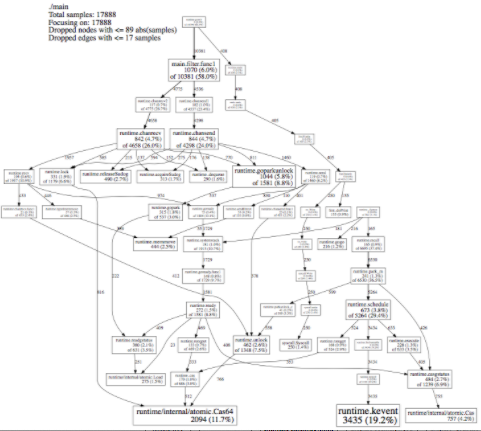dotGraphviz用于画有向图和无向图语言，语法简单。
dot的抽象语法

[ strict ] (graph | digraph) [ ID ] '{' stmt_list '}'


dot支持无向图graph和有向图digraph的绘制，无向图可以理解为没有箭头的有向图，我们直接可以在有向图的edge上使用属性dir=none

graph G1 {
a -- b;
a -- d;
b -- c;
d -- c;
}

digraph G1 {
edge [dir=none];
a -> b;
a -> d;
b -> c;
d -> c;
}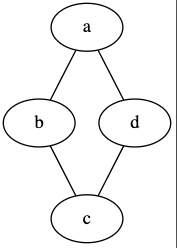### 关键字

• node：节点属性
• edge：连线属性
• graph：图属性，或声明一个无向图时使用
• digraph：有向图
• subgraph：声明子视图，名字要以cluster开头。
• strict：严格模式，用于防止相同的两个节点间使用重复的连线。

## 节点和属性

digraph G1 {
a;
b; c;
"x.y";
}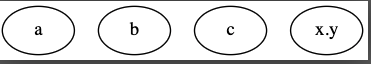### 边

digraph G1 {
a -> b;
a -> c [dir=back];
a -> {d; "x.y"};
}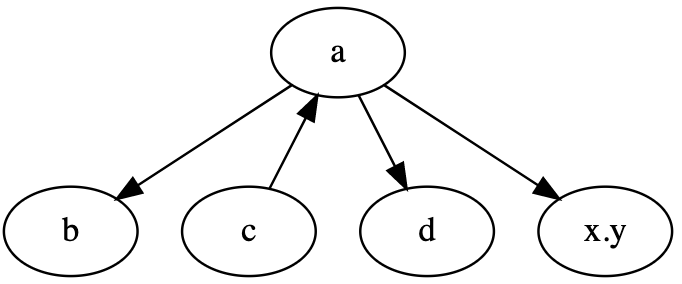### label

dot可以为所有的元素添（graph, node, edge）加label，比如我们的node默认情况下显示的就是他的名字。如果我们想换一个名字可以使用label来进行修改，label不仅支付字符串，而且支持htmlhtml内容要放在要用<>

digraph G {
label = "https://github.com/lpxxn"
n1 [label="hello world"];
a -> b[label=abc];
c [label=<Test<BR /><FONT POINT-SIZE="10">Group: g2</FONT>>; color=orange];
d [shape = record, label="{x|y|z}"]
}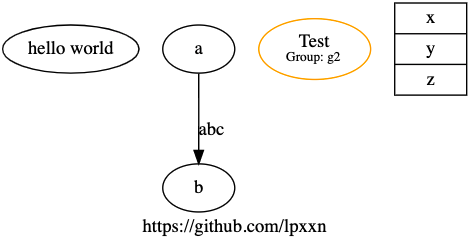### 属性

digraph G1 {
graph[bgcolor=lightblue];
a [color=blue; style=filled; fontcolor=green];
a -> b [color=red; label=A];
edge [color=green]
a -> {c; "x.y"};
}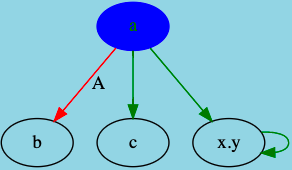graph全局图属性，比如我们把背景颜色变也了淡蓝色，图属性是全局的，除了在graph[]里使用，我们也可以在graph[]外使用，比如控制图的方向rankdir=LR
node全局节点属性，我们可以控制全局的节点属性比如我们可以控制所有节点的形状
edge全局的边属性

digraph G1 {
graph[bgcolor=lightblue];
rankdir=LR
node[shape=box];
a [color=blue; style=filled; fontcolor=green];
a -> b [color=red; label=A; dir=back];
edge [color=green]
a -> {c; "x.y"};
"x.y" -> "x.y";
}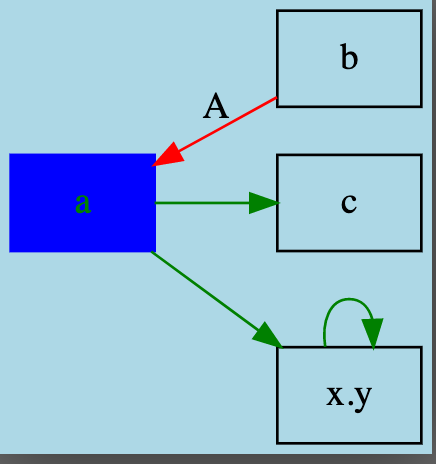### 子视图

digraph G1 {
rankdir=RL;
graph [compound=true];
subgraph cluster_1 {
label = "https://github.com/lpxxn";
edge [dir=none, color=green];
A; B; C;
};
N1 [shape=diamond label=<hello<BR /><FONT POINT-SIZE="10">world</FONT>> color=orange];
subgraph cluster_2 {
label = "https://github.com/lpxxn";
edge [color=red];
D; E;
};
D -> N1 [ltail=cluster_2];
N1 -> B;
E -> C;
}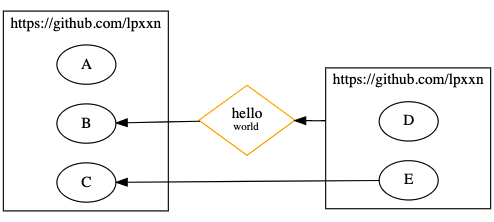### 总结

posted @ 2021-03-29 09:46  li-peng  阅读(1568)  评论(0编辑  收藏  举报